# Quantum Transitions

The following article is from The Great Soviet Encyclopedia (1979). It might be outdated or ideologically biased.

## Quantum Transitions

discontinuous transitions of a quantum system (an atom, a molecule, an atomic nucleus, a solid) from one state to another. The most important are quantum transitions between stationary states that correspond to different energies of the quantum system—the quantum transitions of a system from one energy level to another. During a transition from a higher energy level εk to a lower level the system releases the energy εk–εi and during the reverse transition, absorbs it (see Figure 1).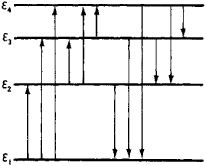Figure 1. Part of the levels of a quantum system: ε1 is the principal level (level with the lowest possible energy) and ε2, ε3, and ε4 are the excited levels. The arrows indicate transitions involving the absorption (directed up) and release (directed down) of energy.

Quantum transitions may be radiative or nonradiative. In radiative transitions the system emits (the transition εk εi) or absorbs (the transition εi → εk) a quantum of electromagnetic radiation—the photon—of energy hv (v is the frequency of radiation and ℏ is the Planck constant), which satisfies the fundamental relation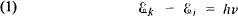(which is the law of conservation of energy for such a transition). Depending on the energy difference between the states of the system in which the quantum transitions take place, photons of radio-frequency, infrared, visible, ultraviolet, and gamma radiation and X-radiation are emitted or absorbed. The aggregate of radiative quantum transitions from lower energy levels to higher ones forms the absorption spectrum of a given quantum system, while the aggregate of the reverse transitions forms its emission spectrum.

In nonradiative quantum transitions, the system acquires or releases energy on interaction with other systems. For example, on colliding with each other and with electrons, the atoms or molecules of a gas may gain energy (be excited) or lose it.

The transition probability, which defines how often a given quantum transition occurs, is the most important characteristic of any quantum transition. It is measured by the number of transitions of a given type in the quantum system under consideration per unit time (1 sec). Therefore, it may assume any values from 0 to ∞ (in contrast to the probability of a single event, which cannot exceed 1). Transition probabilities are calculated by the methods of quantum mechanics.

Quantum transitions in atoms and molecules will be examined below.

Radiative transitions. Radiative quantum transitions may be spontaneous, independent of external influences on the quantum system (the spontaneous emission of a photon), or stimulated, induced by the action of external electromagnetic radiation of a resonance [satisfying relation (1)] frequency v (absorption and stimulated emission of photons). Insofar as spontaneous emission is possible, a quantum system remains at an excited energy level εk for some finite time, and then jumps to some lower level. The mean time period τk that the system remains at the excited level εk is called the lifetime of the energy level. The smaller the τk, the greater the probability of the system’s transition to a state of lower energy. The quantity Ak = 1/τ2, which defines the average number of photons emitted by a single particle (an atom or molecule) per second (τk is expressed in seconds) is called the probability of spontaneous emission from the level εk. For the simplest case of spontaneous transition from the first excited level ε2 to the ground level ε1 the quantity A2 = 1/τ2 defines the probability of this transition; it may be designated A21. Quantum transitions to various lower levels are possible from excited higher levels (see Figure 1). The total number Ak of photons emitted on the average by one particle with an energy εk per second is equal to the total number Aki of photons emitted upon the individual transitions: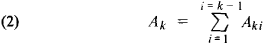that is, the total probability Ak of spontaneous emission from the level εk is equal to the sum of the probabilities Aki of the individual spontaneous transitions εk →εi, the quantity Aki is called Einstein’s coefficient of spontaneous emission upon such a transition. For the hydrogen atom, Aki ≈ (107-108) sec-1.

For induced quantum transitions the number of transitions is proportional to the radiation density pv of the frequency v = (εk — εi)/h that is, to the energy of photons of frequency v per 1 cm3. The probabilities of absorption and stimulated emission are characterized by Einstein’s coefficients Bik and Bki, respectively, which are equal to the number of photons absorbed and stimulated to emission on the average by one particle per second at a radiation density equal to 1. The products Bikpv and Bkipv define the probabilities of stimulated absorption and emission on exposure to external electromagnetic radiation of density pv, and, like Aki are expressed in sec-1.

The coefficients Aki, Bik, and Bki are interconnected by the following relations, which were first derived by Einstein and have been rigorously substantiated in quantum electrodynamics: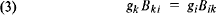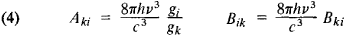where gi(gk) is the degeneration multiplicity of the level εi, (εk), that is, the number of different states of the system that have the same energy εi, (or εk) and c is the velocity of light. For transitions between nondegenerate levels (gi, = gk = 1), Bki = B ik, that is, the probabilities of induced quantum transitions—direct and reverse—are identical. If one of the Einstein coefficients is known, then the others can be determined from relations (3) and (4).

The probabilities of radiative transitions are different for different transitions and depend on the properties of the energy levels εi, and εk between which the transition takes place. The more strongly the electric and magnetic properties of the quantum system that are characterized by its electric and magnetic moments change upon transition, the greater the probabilities of quantum transitions. The possibility of radiative quantum transitions between the energy levels εi, and εk with assigned characteristics is defined by the selection rules. (For greater detail, seeRADIATION, ELECTROMAGNETIC.)

Nonradiative transitions. Nonradiative quantum transitions are also characterized by the probabilities of the corresponding transitions Cki and Cik—the average number of processes of release and acquisition of energy εk — εi, per 1 sec, calculated for one particle with an energy εk (for the energy release process) or an energy ε, (for the energy acquisition process). If both radiative and nonradiative quantum transitions are possible, then the total transition probability is equal to the sum of the transition probabilities of both types.

It is essential to take into account nonradiative quantum transitions when the transition probability is of the same order or greater than the corresponding quantum transition involving radiation. For example, if spontaneous radiative transition to the ground level ε1 from the first excited state ε2 is possible with a probability A21 and nonradiative transition to the same level is possible with a probability C21 then the total transition probability will be equal to A21 + C2 the energy level lifetime is equal to τ’2 = 1/(A21 + C21) instead of τ2 = 1/A2 in the absence of a nonradiative transition. Thus, the energy level lifetime decreases because of nonradiative quantum transitions. When C21A21, the time τ2’ is very small in comparison with τ2, and the majority of particles will lose their excitation energy ε2 — ε1 in nonradiative processes—the quenching of spontaneous emissions will take place.

M. IA. EL’IASHEVICH

References in periodicals archive ?
QED works and radioactive decay has a exponential half life, due to Poisson in time quantum transitions.
[We note that dipole matrix elements for nS [right arrow] 1S quantum transitions are zero.
Red and green lasers irradiate them to excite a series of quantum transitions that yield fluorescent light.
It is shown that the quantization is actually destroyed, in general, by a non-inertial motion in the presence of external forces, in the sense that such a motion may produce quantum transitions. Examples are given for a massive scalar field and for photons.
It follows that an observer in a non-uniform translation might see quantum transitions between the states of a relativistic
The particle exhibits quantum transitions, which make its quantum state undetermined.
It is the hamiltonian (14) which is subjected to quantization in that case, so we may have quantum transitions between the states of the particle, providing these states do not conserve the angular momentum.
The non-uniform translation in the left side of equation (50) gives now quantum transitions.
The qualitative conclusions derived above for equation (44), as regards the quantum transitions produced by the non-uniform translation, remain valid, though, we have now a language of fields.
This can be most conveniently expressed in terms of photons which suffer quantum transitions.
Systematic calculations can now be performed within the perturbation theory, and we can see that quantum transitions between the photonic states may appear, starting with the hg-order of the perturbation theory.

Site: Follow: Share:
Open / Close